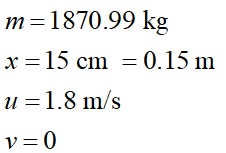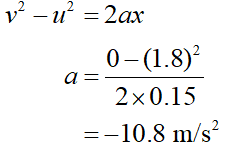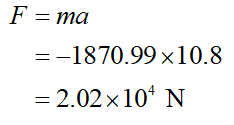# Bumpers are built with springs that compress to provide a stopping force without, hopefully, denting the metal. Bumpers on cars are not of much use in a collision. To see why, calculate the magnitude of average force (to the nearest Newton) a bumper would have to exert if it brought a 1,870.99-kg car (a so-called compact model) to a rest in 15 cm when the car had an initial speed of 1.8 m/s.

Question
74 views
Bumpers are built with springs that compress to provide a stopping force without, hopefully, denting the metal. Bumpers on cars are not of much use in a collision. To see why, calculate the magnitude of average force (to the nearest Newton) a bumper would have to exert if it brought a 1,870.99-kg car (a so-called compact model) to a rest in 15 cm when the car had an initial speed of 1.8 m/s.

check_circle

star
star
star
star
star
1 Rating
Step 1

It is given thatStep 2

To find the force, we need to find the acceleration. Using newton’s third equation of motion,Step 3

Force is given as,...

### Want to see the full answer?

See Solution

#### Want to see this answer and more?

Solutions are written by subject experts who are available 24/7. Questions are typically answered within 1 hour.*

See Solution
*Response times may vary by subject and question.
Tagged in